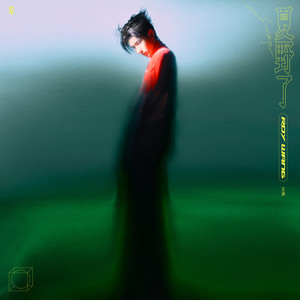疯人公园-王源

• 高清播放

歌词

Let it go Let it go Let it go yeah

Let you know Let you know yeah

Let it go Let it go Let it go yeah

Let you know Let you know yeah

Let it go Let it go Let it go yeah

Let you know Let you know yeah

Let it go Let it go Let it go yeah

Let you know Let you know yeah

Let it go Let it go Let it go yeah

Let you know Let you know yeah

Let it go Let it go Let it go yeah

Let you know Let you know yeah